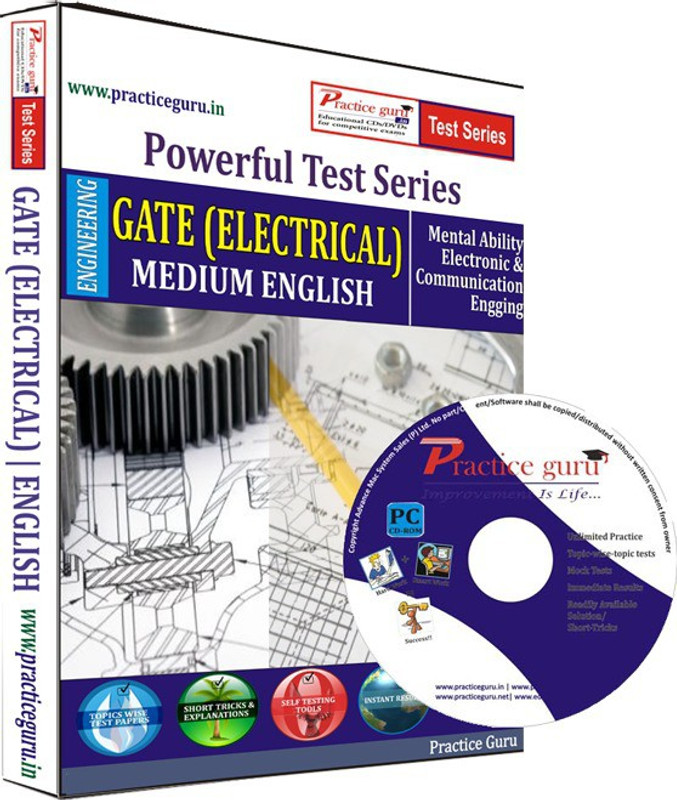# Practice Guru GATE - Electrical Test Series(CD)

## Product Offers

• 5% off on Visa Cards for COD, New users
• 10% off* with Axis Bank Buzz Credit Card

## Key Specification

• Educational Board: CBSE
• Subjects: Physics, Chemistry, Mathematics
• Classes: PUC-II
• Available in CD
• Disks Included: 1
• Subjects
Physics, Chemistry, Mathematics
• Subscription Validity
1 Year
• Sales Package
Educational Media
• Model Name
GATE - Electrical Test Series
• Classes
PUC-II
• Num Of Disks
1
• Model Id
FP-21
• Education Board
CBSE
• Brand
Practice Guru
• Processor
Intel Pentium, Celeron, XeonTM
• Hard Drive
20 GB to 5 GB
• Operating System
Windows
• Memory
531 MB or more

"Engineering Mathematics: Linear Algebra: Matrix Algebra, Systems of linear equations, Eigen values and Eigen vectors Calculus: Mean value theorems, Theorems of integral calculus, Evaluation of definite and improper integrals, Partial Derivatives Differential equations: First order equation (linear and nonlinear), Higher order linear differential equations with constant coefficients, Method of variation of parameters Complex variables: Analytic functions, Cauchy's integral theorem and integral formula, Taylor's and Laurent' series, Residue theorem, Solution integrals. Probability and Statistics: Sampling theorems, Conditional probability, Mean, Median, Mode and standard deviation Numerical Methods: Solutions of non-linear algebraic equations, Single and multi-step methods for differential equations, Transform Theory: Fourier transform, Laplace transform, Z-transform Electrical Engineering: Electric Circuits and Fields: Network graph, KCL, KVL, Node and mesh analysis, Transient response of dc and ac networks; sinusoidal steady-state analysis, Resonance, Basic filter concepts; ideal current and voltage sources, The venin?????s, Norton's and Superposition and Maximum Power Transfer theorems, Two-port networks, Three phase circuits; Gauss Theorem, Electric field and potential due to point, Line, Plane and spherical charge distributions; Ampere's and Biot-Savart's laws; inductance; dielectrics; capacitance. Signals and Systems: Representation of continuous and discrete-time signals; shifting and scaling operations; linear, time-invariant and causal systems; Fourier series representation of continuous periodic signals; sampling theorem; Fourier, Laplace and Z transforms Electrical Machines: Single phase transformer -equivalent circuit, Pastor diagram, Tests, Regulation and efficiency; three phase transformers-connections, Parallel operation, Auto-transformer, Energy conversion principles, DC machines ?????????types, Windings, Generator characteristics, Armature reaction and commutation, Starting and speed control of motors; three phase induction motors ?????????principles, Types performance, characteristics, Starting and speed control; single phase induction motors; synchronous machines ?????????performance, Regulation and parallel operation of generators, Motor starting, Characteristics and applications; servo and stepper motors. Power Systems: Basic power generation concepts; transmission line models and performance; cable performance insulation; corona and radio interference; distribution systems; per-unit quantities; bus impedance and admittance matrices; load flow; voltage control; power factor correction; economic operation; symmetrical components; fault analysis; principles of over-current differential and distance protection; solid state relays and digital protection; circuit breakers; system stability concepts swing curves and equal area criterion; HVDC transmission and FACTS concepts Control Systems: Principles of feedback; transfer function; block diagrams; steady-state errors; Rough and Inquest techniques; Bode plots; root loci; lag lead and lead-lag compensation; state space model; state transition matrix, controllability and observe ability Electrical and Electronic Measurements: Bridges and potentiometers; PMMC, Moving iron, Dynamometer and induction type instruments; measurement of voltage, Current Power energy and power factor; instrument transformers; digital voltmeters and millimeters; phase time and frequency measurement; Q-meters; oscilloscopes; potentiometric recorders; error analysis Analog and Digital Electronics: Characteristics of diodes, BJT, FET; amplifiers ?????????biasing equivalent circuit and frequency response; oscillators and feedback amplifiers; operational amplifiers -characteristics and applications; simple active filters; VCOs and timers; combinational and sequential logic circuits; multiplexer; Schmitt trigger; multi-vibrators; sample and hold circuits; A/D and D/A converters; 8-bit microprocessor basics, Architecture, Programming and interfacing Power Electronics and Drives: Semiconductor power diodes, Transistors, thrusters, traces, GTOs, MOSFETs and IGBTs -static characteristics and principles of operation; triggering circuits; phase control rectifiers; bridge converters -fully controlled and half controlled; principles of choppers and inverters; basis concepts of adjustable speed dc and ac drives + MOCK TESTS for GATE - GATE - Electrical"